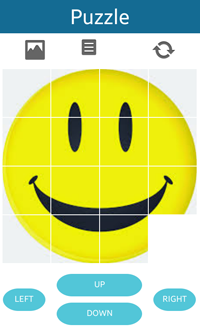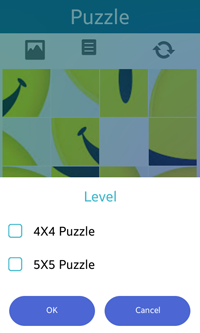# Puzzle Sample OverviewThe Puzzle sample application demonstrates how to implement a complex view using recursive composition of the standard EFL components.

The following figure illustrates the main view of the Puzzle application in its normal state.

Figure: Puzzle main view## Implementation

To create the Puzzle:

1. Create the layout for the Puzzle preview.

The puzzle_create() function creates the main layout of the Puzzle application.

```void
puzzle_create(appdata_s *data)
{
_D("Puzzle_create");
ret_if(!data);
// Make a 6X6 image object
for (i = 0; i < 37; i++)
{
{
}
}

// Divide the size of a real image into a puzzle

// Divide the size of the puzzle of the screen into a PIECE

for (y = 0; y < PIECE; y++)
{
for (x = 0; x < PIECE; x++)
{
y*extract_region_height, extract_region_width, extract_region_height);

if (y == 3 && x == 3)
{

}

}
}
}
```
2. Move the piece and match the picture.

The move_***_cb() function moves the piece to ***. When moving the piece to ***, if there is a piece already, this function returns with no action.

```int
move_puzzle_to_right(void *data)
{
retv_if(!data, 0);

int x1, y1, w1, h1;
int x2, y2, w2, h2;
int temp;
// There is a puzzle
if (!(white_piece%size))
{
return SAMPLE_APP_ERROR_OK;
}

// Exchange the position

{
}

return 1;
}
```
3. Complete the puzzle.

If the image object is located in its original position, the puzzle_correct_cb() function is called to show the original picture of the puzzle.

```void
puzzle_correct_cb(appdata_s *data)
{
ret_if(!data);

{
{
return;
}
}
// Make a popup for congratulations
elm_popup_align_set(popup, ELM_NOTIFY_ALIGN_FILL, 1.0);

elm_object_part_text_set(popup, "title,text", "Correct!!");
evas_object_size_hint_weight_set(popup, EVAS_HINT_EXPAND, EVAS_HINT_EXPAND);

evas_object_size_hint_weight_set(layout, 0, 0);
evas_object_size_hint_align_set(layout, 0, EVAS_HINT_FILL);
elm_object_content_set(popup, layout);

// Show the original picture of the puzzle
elm_image_aspect_fixed_set(img, EINA_FALSE);

evas_object_size_hint_min_set(img, 400, 400);
evas_object_size_hint_max_set(img, 400, 400);
elm_object_part_content_set(layout, "elm.swallow.content", img);

// Make a popup delete button
elm_object_style_set(btn, "popup");
elm_object_text_set(btn, "OK");
elm_object_part_content_set(popup, "button1", btn);
evas_object_show(popup);

}
```
4. Select the level of the puzzle.

Figure: Puzzle levelsThe user can select the level of the puzzle. Select a 4X4 puzzle or a 5X5 puzzle. To change the number of pieces, the puzzle_change() function is called. It works in the same way as the puzzle_create() function, but the value of PIECE is different.

```void
_level_button_cb(void *data, Evas_Object *obj, void *event_info)
{
ret_if(!data);

Evas_Object *popup = NULL;

{
{
_E("Failed to change puzzle");
}
}